#### FM442: Quantitative Methods for Finance and Risk Analysis

Jon Danielsson
Michaelmas Term 2021

## Practice exam questions on Risk Measures¶

### Question 1¶

Write down the mathematical definition of VaR (Value-at-Risk), and derive ES (Expected Shortfall).

#### Solution¶

VaR is a quantile on the distribution of P/L (profit and loss), so to write down the mathematical definition, we need to use $Q$, the random variable for the P/L on an investment portfolio. The realized value is $q$. So, we can denote the density of the P/L distribuition by $f_q(\cdot)$, and define VaR by:

$$\textrm{Pr}\ [Q\ \leq -\textrm{VaR}(p)] = p$$

or,

$$p = \int_{-\infty}^{-\textrm{VaR}(p)}f_q(x)dx$$

Expected shortfall is the expected loss conditional on VaR being violated:

$$\textrm{ES} = - E[Q|Q ≤ - \textrm{VaR(p)}]$$

If we write it down in its integral form:

$$\textrm{ES} = \int_{-\infty}^{-\textrm{VaR}(p)} x f_{\textrm{VaR}}(x)dx$$

The tail density, $f_{\textrm{VaR}}(\cdot)$ is given by:

$$1 = \int_{-\infty}^{-\textrm{VaR}(p)} f_{\textrm{VaR}}(x)dx\ =\ \frac{1}{p}\ \int_{-\infty}^{-\textrm{VaR}(p)} f_{q}(x)dx$$

### Question 2¶

What is a coherent risk measure?

#### Solution¶

Consider two real-valued random variables: $X$ and $Y$. A function $\varphi(\cdot): X,Y \rightarrow \mathbb{R}$ is called a coherent risk measure if it satisfies for $X$, $Y$, and constant $c$:

$\textrm{(a) Monotonicity}$ $$\textrm{if}\ X, Y \in V\ \textrm{and}\ X \leq Y,\ \textrm{then},\ \varphi(X) \geq \varphi(Y)$$

$\textrm{(b) Subadditivity}$ $$\textrm{if}\ X, Y, (X+Y) \in V,\ \textrm{then},\ \varphi(X+Y) \leq \varphi(X) + \varphi(Y)$$

$\textrm{(c) Positive homogeneity}$ $$\textrm{if}\ X \in V\ \textrm{and}\ c > 0,\ \textrm{then},\ \varphi(cX) = c\varphi(X)$$

$\textrm{(d) Translation invariance}$ $$\textrm{if}\ X \in V\ \textrm{and}\ c \in \mathbb{R},\ \textrm{then},\ \varphi(X + c) = \varphi(X) - c$$

### Question 3¶

Suppose you own two assets, A and B, with payoffs that are independent of each other. Each asset returns either 0 with probability 0.9, or -100 with probability 0.1. Is VaR(5%) sub-additive?

#### Solution¶

First let's consider only one of the assets, say asset A. With probability 10%, losses would be 100, and with probability 90%, losses would be 0. We can directly calculate the VaR of the single asset as:

VaR for A is: $$\textrm{VaR}^{5\%}(A) = 100$$ $$\textrm{VaR}^{15\%}(A) = 0$$

Now let's consider a owning one unit of asset A and one unit of asset B, so returns are (0.5A + 0.5B). We have a portfolio with two assets with independent payoffs. Each asset has two possible realizations, which means our portfolio has four possible payoffs. We can build a table with these payoffs and their corresponding probabilities:

Asset A Asset B Portfolio Return Probability
0 0 0 (0.9) * (0.9) = 0.81
-100 -100 -200 (0.1) * (0.1) = 0.01
0 -100 -100 (1 - 0.81 - 0.01) / 2 = 0.09
-100 0 -100 (1 - 0.81 - 0.01) / 2 = 0.09

If we order the possible portfolio returns and look for the 5% quantile, it will be the case where one asset incurs in a loss and the other does not. so $\textrm{VaR}^{5\%}(A+B) = 100$.

Hence:

$$\textrm{VaR}^{5\%}(A+B) = 100 \leq \textrm{VaR}^{5\%}(A) + \textrm{VaR}^{5\%}(B) = 200$$

### Question 4¶

Consider the asets in the last question. Is VaR(15%) sub-additive?

#### Solution¶

If we are considering a single asset, there is only a 10% probability of a loss of -100. This means that any VaR with probability larger than 10% will be equal to zero:

$$\textrm{VaR}^{15\%}(A) = \textrm{VaR}^{15\%}(B) = 0$$

For the case of the portfolio, ordering the possible returns in the table from Question 3 shows us that with a 15% probability, we would still be in the situation where one asset incurs in losses while the other does not, which means:

$$\textrm{VaR}^{15\%}(A + B) = 100$$

Since the VaR of the portfolio is larger than the sum of the individual VaRs, VaR(15%) fails to meet subadditivity.

### Question 5¶

Give one example of a traded asset that could lead to a sub-additivity violation of VaR, carefully explaining why the payoff structure of this asset would lead to that conclusion.

#### Solution¶

An asset that most of the time has a return of zero, but occasionally very negative. This could be the exchange rate of a country with a peg, that occasionally devalues, short deep out of the money option or junk bonds.

The payoff structure of these type of assets can resemble the one discussed in Question 3, which means that by diversifying, you can have a positive VaR for a probability that would give you a VaR of 0 if you were considering a single asset.

### Question 6¶

Suppose you own 1 million USD worth of both stocks A and B. Stock A is a small cap stock, with a market capitalization of 3 million, while stock B is a large cap stock, with a market capitalization of 3 billion. As a consequence, one of the axioms of a coherent risk measure is likely to be violated for VaR on one of the stocks but not the other. Which stock is it and why would that be the case?

#### Solution¶

This question is about the positive homogeneity property of risk measures: $$c \times Risk(A) = Risk(c\times A)$$ for an asset $A$ and constant $c$.

It is very different owning 1 million USD worth of a stock with a total market capitalization of 3 million, or a stock with total market capitalization of 3 billion. In the first case, we have a big influence on the price of the stock. If we decide to sell all our holdings, the price will go down as we do. This means we will not be getting the same amount of money for each dollar worth of stock we sell. In the case of the large capitalization company, our holdings are a small share of the total (less than 1%), so it is unlikely that any transaction we do will have a large impact on the stock price.

So, positive homogeneity is likely to hold for the big capitalization firm, but not for the small one.

### Question 7¶

State the three steps in VaR calculations.

#### Solution¶

(a) To specify probability $p$. Commonly used are VaR levels of 1% - 5%, but it is mainly determined by how the risk managers wishes to interpret the VaR number.

(b) To decide holding periods, commonly 1 day, but it can vary depending on different circumstances.

(c) Identification of probability distribution of the profit and loss of the portfolio.

## Estimating VaR and ES with historical simulation - Univariate¶

Historical simulation is a non-parametric method to implement risk measures. It assumes that the past is a sufficient predictor for the future, so in other words, that "history repeats itself". It uses the quantiles of the empirical disitribution of returns. The procedure to implement it for estimating VaR is:

• Decide on a probability, $p$, e.g. 1%
• Have a sample of returns, $y$, with length $T$, e.g. 1000 days (for multi-assets, you can treat $y$ as the portfolio returns)
• Sort the $y$ from smalles to largest, call that $ys$
• Take the $(T \times p)^{th} = (1000 \times 0.01)^{th}$ smallest value of $ys$, call that $ys_{T \times p} = ys_{10}$
• If you own one stock, and $P_{t-1}=1$, then VaR is the $10^{th}$ smallest return, i.e. $$VaR_t = -ys_{10}$$
• Otherwise, you have to multiply that by the number of stocks you own, and their $t-1$ price: $$VaR_t = -ys_{10} P_{t-1} \textrm{number of stocks}$$

To implement it for ES:

• Take the mean of all observations that violate VaR
• For example, for the previous case: $$\textrm{ES} = \frac{1}{10} \sum_{i=1}^{10} ys_i$$

#### Portfolio Value¶

We will use the term portfolio value to denote the monetary value of a portfolio. For example, if we own 100 stocks, each worth 10 USD, the value of our portfolio is of 1000 USD. We can extend this idea to a portfolio with different assets. If we have 5 stocks of A, each worth 10 USD, and 20 stocks of B, each worth 2 USD, the value of our portfolio is: (5)(10) + (20)(2) = 90 USD.

### Implementing VaR and ES for General Electric¶

We will use historical simulation to esimate VaR and ES for the returns of General Electric:

In :
# Implementing HS to estimate VaR and ES

# Extract the returns for GE
y <- Y$GE # View them head(y)  1. 0.034289343141517 2. -0.00187475625782325 3. -0.00564490252545496 4. -0.00947878204978265 5. 0.00569773702368828 6. -0.0210530687723584 In : # Plot the returns plot(y, type = "l", main = "Returns for GE")In : # Specify the probability p p <- 0.05 # Assume we have a portfolio value of 1000 USD portfolio <- 1000 # Sort the values in y using sort() ys <- sort(y) # Plot them plot(ys, type = "l", main = "Sorted returns for GE", las = 1)In : # Number of observations n <- length(y) # Get the 5% quantile by multiplying the length times p n * p  377.95 Since this number is not an integer, it is a good practice to round it up. If we round it down, we are not meeting the required probability, since the element in the 377th position has a quantile lower than 5%. By rounding up and using the element in the 378th position, we guarantee to be covering the 5% quantile. We can use the function ceiling() for this: In : # Round up quant <- ceiling(n*p) quant  378 Now we search for the 387th element in the sorted vector ys: In : # Find the 378th element in ys ys[quant]  -0.0265667927693648 We can visually see this in the plot for the sorted list: In : # Visually finding the 5% quantile plot(ys, type = "l", main = "Sorted returns for GE", las = 1) # Adding the segments segments(x0 = quant, y0 = -0.5, y1 = ys[quant], lty = "dashed", lwd = 2, col ="red") segments(x0 = -1000, y0 = ys[quant], x1 = quant, lty = "dashed", lwd = 2, col ="red") # Adding ticks axis(1, at = quant, label = quant) axis(2, at = ys[quant], label = round(ys[quant],3), las = 1)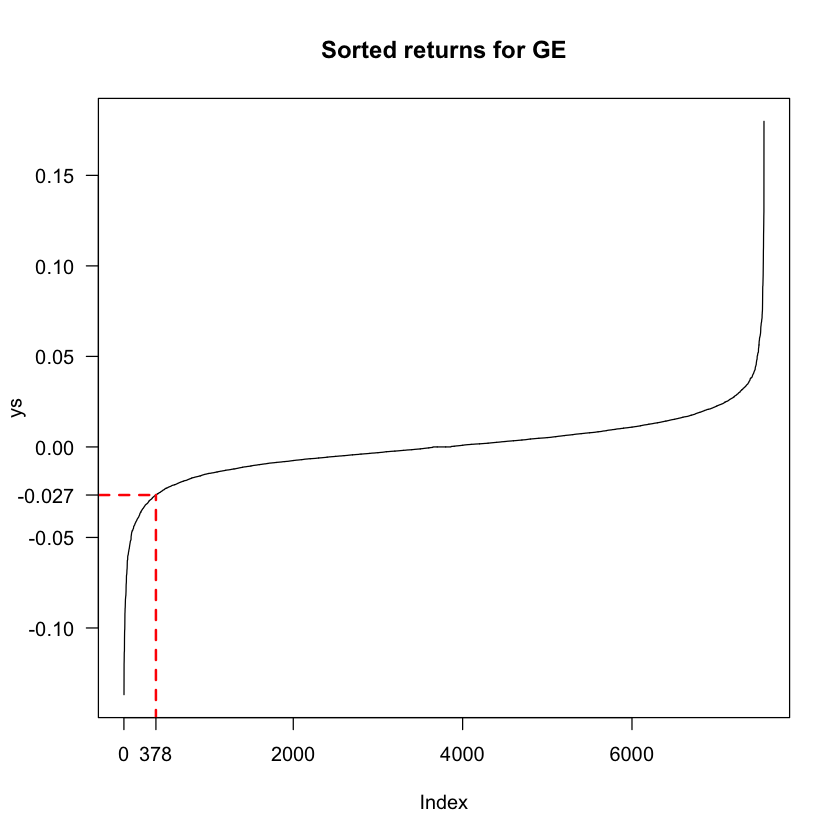In order to calculate VaR, we multiply this return by the portfolio value, with a minus sign in front since it is a loss: In : # Use it to calculate VaR, scaling for the portfolio value VaR <- -ys[quant] * portfolio VaR  26.5667927693648 To calculate the ES, we take the mean of returns conditional on VaR being violated, and scale this to the portfolio size. In other words In : # For ES, we get the mean of observations up to the 5th quantile ES <- -mean(ys[1:quant]) * portfolio ES  42.9323990472894 In : # Report our findings: cat("We have", length(y), "observations on GE", "\n", p , "VaR is", VaR, "and ES is", ES, "on portfolio worth$", portfolio, "\n")

We have 7559 observations on GE
0.05 VaR is 26.56679 and ES is 42.9324 on portfolio worth $1000  A direct way to find the quantile of an empirical distribution is using the quantile() function on the returns. But bear in mind that if the quantile index is not integer, it will output a weighted average between the rounded-up and rounded-down values. By calling quantile(y,0.05) we see a small difference: In : # Doing HS with the quantile() function quant2 <- quantile(y,0.05) quant2 # VaR VaR <- -quant2*portfolio cat("5% VaR is:", VaR)  5%: -0.0265603231787511 5% VaR is: 26.56032 ## Estimating VaR and ES with historical simulation - Multivariate¶ The multivariate extension to HS is quite straightforward. We use the matrix of returns and a vector of weights to create a time series vector of portfolio returns, and treat it as if it were a single asset. In this section we will estimate VaR through HS for General Electric and Citigroup: In : # HS for GE and C # Get the returns from GE and C and transform into a matrix y <- as.matrix(Y[,c("GE", "C")]) # Create a vector of portfolio weights, here we will split the stocks halfway w <- c(0.5, 0.5) # Multiply the matrix y (dimension Tx2) by the vector w (dimension 2x1) # Output yp is portfolio returns, dimension (Tx1) yp <- y %*% w head(yp)   0.0322647 0.00540522 -0.00916501 -0.000501898 0.00705066 -0.0168423 In : # Plot the portfolio returns plot(Y$date, yp, main = "Portfolio returns for GE and C", type = "l", las = 1, lwd = 2, col = "red")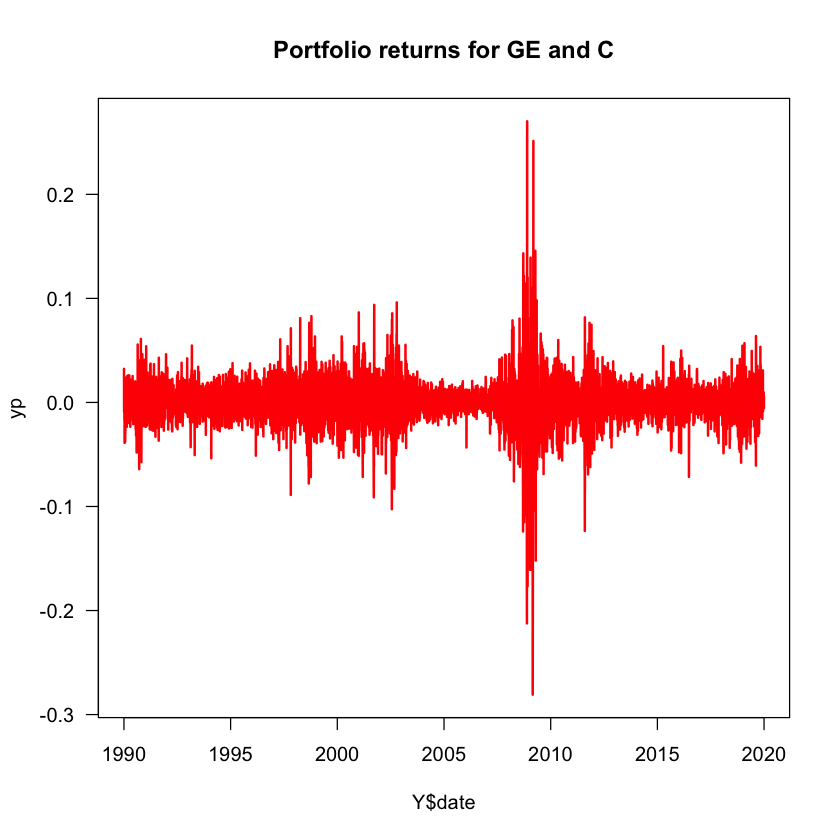In :
# Get VaR and ES

# Sort the vector
ys <- sort(yp)

# Get the length
n <- length(ys)

# Get the position of 5% quantile
quant <- ceiling(n*p)

# VaR
VaR <- -ys[quant]*portfolio

# ES
ES <- -mean(ys[1:quant]) * portfolio

cat("We have", length(ys), "observations on the portfolio", "\n",
p , "VaR is", VaR, "and ES is", ES, "on portfolio worth $", portfolio, "\n")  We have 7559 observations on the portfolio 0.05 VaR is 27.43663 and ES is 47.02372 on portfolio worth$ 1000


## Historical simulation VaR with different estimation windows¶

### Out-of-sample forecasting and estimation windows¶

We usually use a fixed estimation window to do a one-day-ahead VaR forecast recursively. For example, we can the data from day 1 to day 1000 to forecast VaR for day 1001. This is an out-of-sample forecast, since the data we are using only comprises days 1 to 1000, but we are doing a forecast for day 1001, which is not in our sample. Then we will move one period ahead and use data from day 2 to day 1001 to forecast VaR for day 1002 and so on. The estimation window, denoted by $W_E$ is how many days we include in the sample to forecast for one day ahead. In the example just mentioned, the estimation window is 1000 days.

Assume we have a total of $T$ data points. After we are doing with this recursive one-day-ahead forecasting, we will have made $T-W_E$ forecasts.

### Implementation¶

Let's work again with the returns of General Electric and use a fixed estimation window of 1000 days. We will need to implement a for-loop to get the VaR estimation for each day between days 1001 and the length of the dataset.

In :
# Historical simulation with fixed estimation window
y <- Y$GE window <- 1000 p <- 0.05 n <- length(y) portfolio_value <- 1000 # Initialize and empty vector to hold VaR forecasts VaR <- vector(length = (n - window)) # Loop to calculate one VaR per day for (i in 1:(n-window)) { ys <- sort(y[i:(i+window)]) quant <- ceiling(p*length(ys)) VaR[i] <- -ys[quant]*portfolio_value }  In : # Plot it plot(VaR, type = "l", main = "VaR HS with Estimation Window 1000", col = "red", las = 1, ylab = "USD")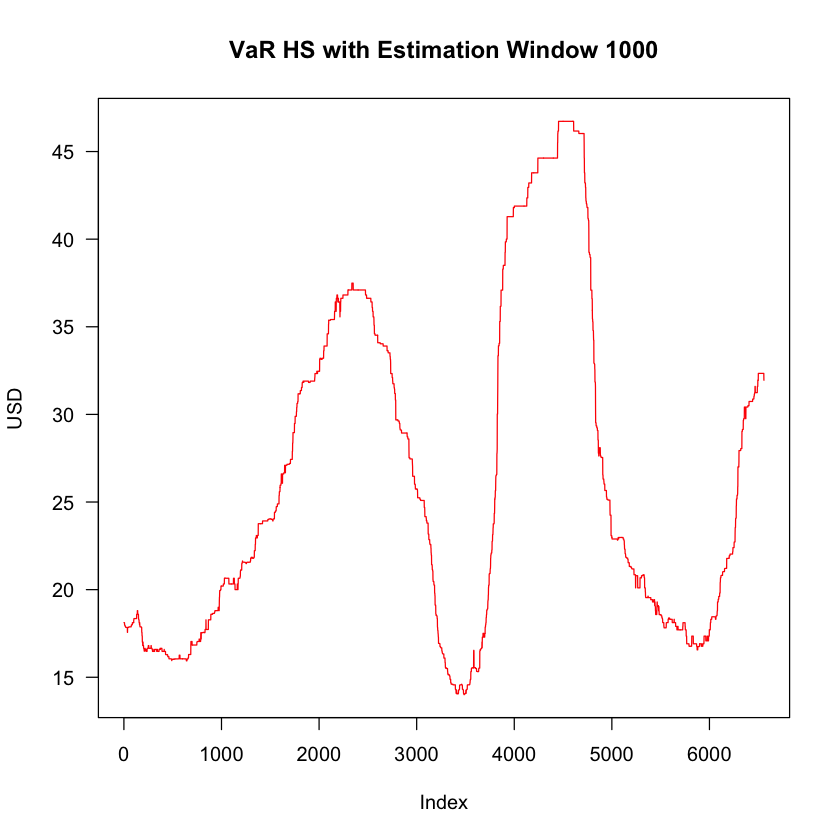In : # We should add the dates in the x-axis # Notice we have to exclude the first 1000 days dates_var <- Y$date[-1:-1000]

# Plot again
plot(dates_var, VaR, type = "l", main = "VaR HS with Estimation Window 1000",
col = "red", las = 1, ylab = "USD", xlab = "Date")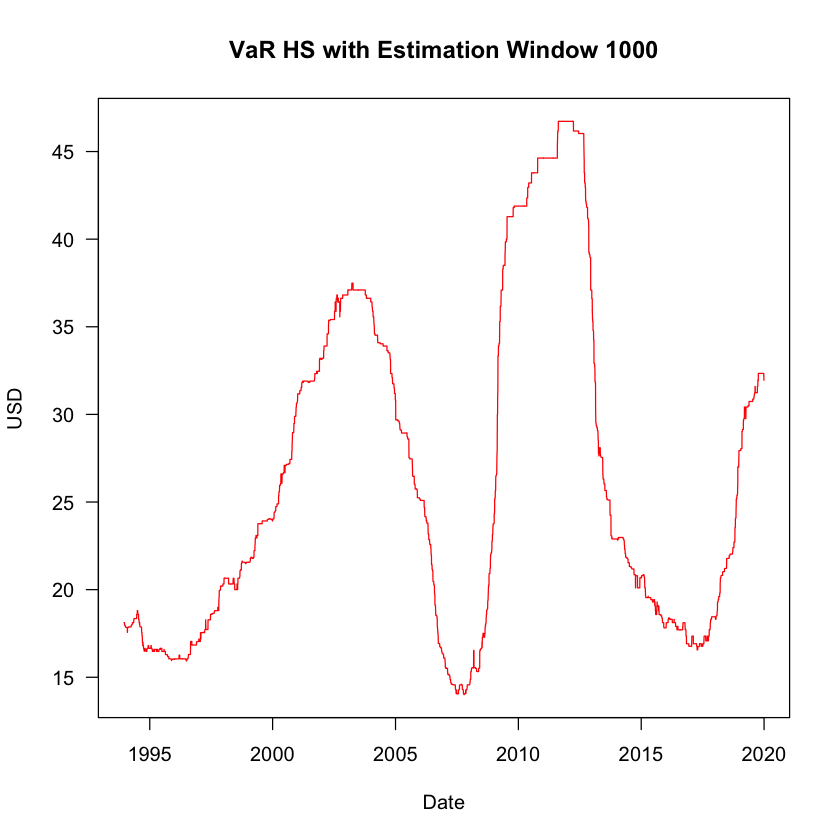### Different Estimation Windows¶

We have used a fixed estimation window of 1000 days. To see the effect of changing the estimation window, we will reproduce this exercise using estimation windows of 100, 500, 1000 and 5000 days:

In :
windows <- c(100, 500, 1000, 5000)
which(windows == 100)

1
In :
# Using four different estimation window sizes
windows <- c(100, 500, 1000, 5000)

# Create an empty data frame to fill with the forecasts
HS <- data.frame(HS100 = numeric(),
HS500 = numeric(),
HS1000 = numeric(),
HS5000 = numeric())

# Do a loop for every element of windows
for (window in windows) {

# Perform a daily HS VaR
for (i in 1:(n-window)) {

# Sort the returns for the respective estimation window
ys <- sort(y[i:(i+window)])

# Get the quantile
quant <- ceiling(p*length(ys))

# Allocate the result to the corresponding column of HS
# Use which() to find the index of the window and allocate to that column
column <- which(windows == window)
HS[i+window, column] <- -ys[quant]*portfolio_value
}
}


Now that we have implemented HS VaR for four different estimation windows, we can compare them. We need to be careful in this step because each model has a different number of forecasts, given that the number of observations used is different. We should always focus on the time frame of the most restrictive model, in this case, the one using an estimation window of 5000 observations.

Let's plot all of them together:

In :
# Plotting the forecasts
plot(HS$HS100, main = "HS with different estimation windows", ylab = "VaR in USD", xlab = "Date", type = "l", col = "red", lwd = 2) lines(HS$HS500, col = "blue", lwd = 2)
lines(HS$HS1000, col = "green", lwd = 2) lines(HS$HS5000, col = "black", lwd = 2)

legend("topright", legend = names(HS), lty = 1, col = c("red", "blue", "green", "black"))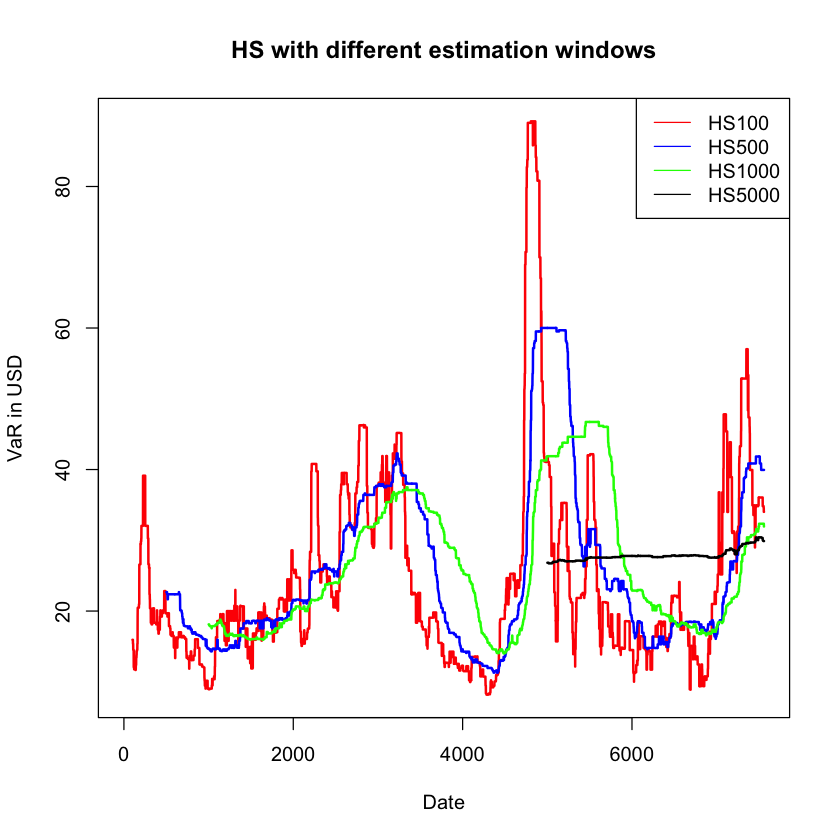For a better comparison, let's restrict our attention to the time frame where we have forecasts for each model. This consists of the length of the data minus the largest estimation window. Also, we will add dates:

In :
# Subset HS to keep only the observations that fit the most restrictive estimation window
HS <- HS[(max(windows)+1):n,]
# Extract the dates to use
dates <- Y$date[(max(windows)+1):n] # Plotting them all together plot(dates, HS$HS100, main = "HS with different estimation windows", ylab = "VaR in USD",
xlab = "Date", type = "l", col = "red", lwd = 2)
lines(dates,HS$HS500, col = "blue", lwd = 2) lines(dates,HS$HS1000, col = "green", lwd = 2)
lines(dates,HS\$HS5000, col = "black", lwd = 2)

legend("bottomright", legend = names(HS), lty = 1, col = c("red", "blue", "green", "black"))